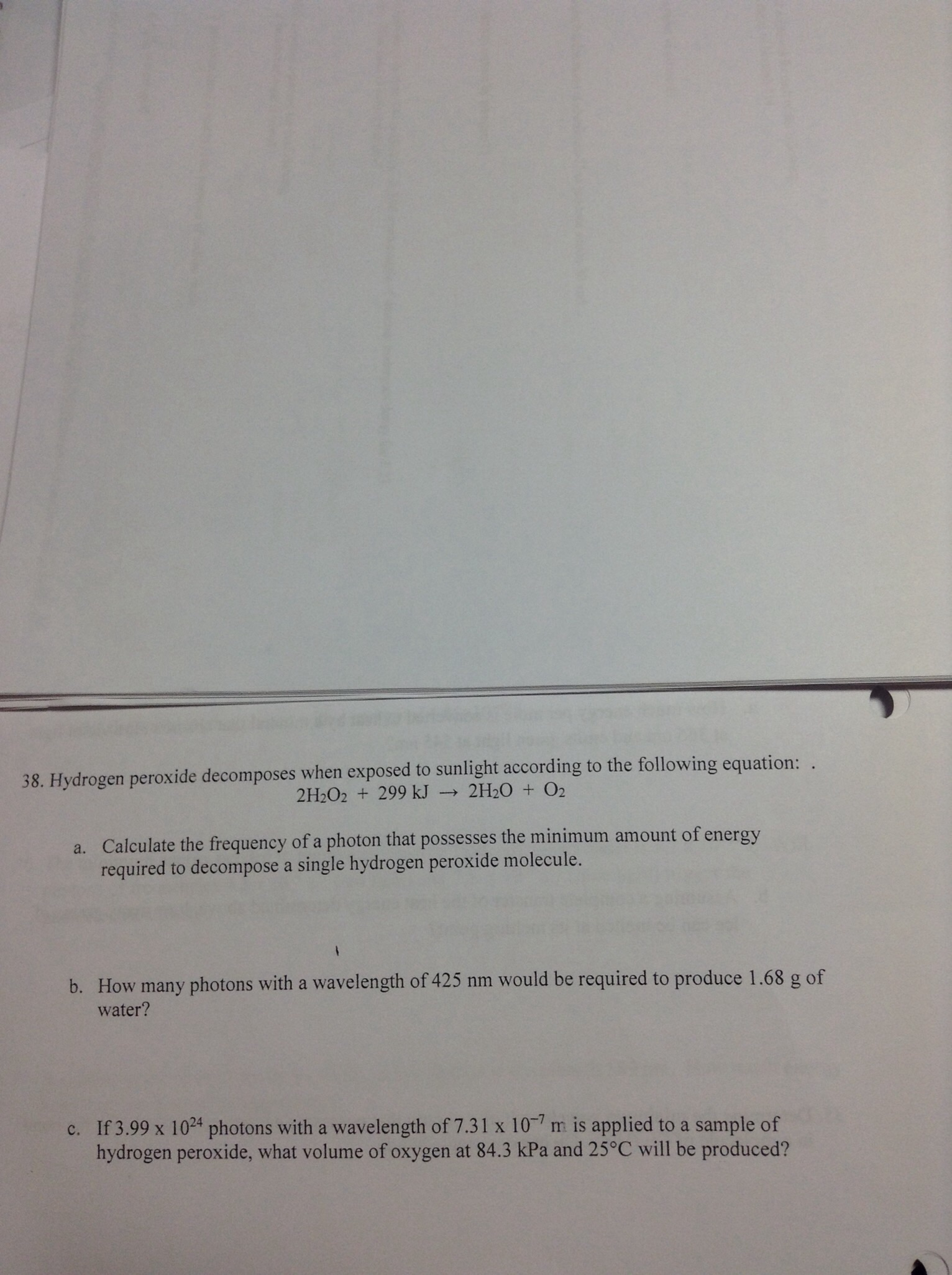# Hydrogen peroxide decomposes when exposed to sunlight according to the following equation: 2H2O2 + 299 kJ → 2 H2O + O2. (a) Calculate the frequency of a photon that possesses the minimum amount of energy required to decompose a single hydrogen peroxide molecule. (b) How many photons with a wavelength of 425 nm would be required to produce 1.68 g of water? (c) If 3.99x10^24 photons with a wavelength of 7.31x10^-7 m is applied to a sample of hydrogen peroxide, what volume of oxygen at 84.3 kPa and 25°C will be produced?